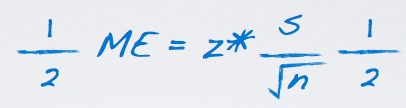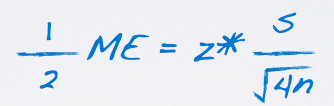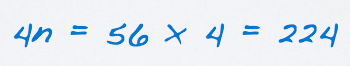### Introduction

In this section, we are going to work with confidence intervals. Specifically, we are going to learn how to calculate the required sample size to achieve a certain margin of error.

We are also going to discuss sample size vs. accuracy.

Given a target margin of error, confidence level, and information on the variability of the sample (or population), we can determine the required sample size to achieve the desired margin of error.

We do this by plugging known values into the equation of the margin of error and then rearranging things to solve for the unknown sample size:### Example

A group of researchers want to test the possible effect of an epilepsy medication taken by pregnant mothers on the cognitive development of their children. As evidence, they want to estimate the IQ scores of three-year-old children born to mothers who where on this medication during pregnancy.

Previous studies suggest that the SD of IQ scores of three-year-old chilren is 18 points. How many such children should the researchers sample in order to obtain a 90% confidence interval with a margin of error less than or equal to 4 points?

So, we know:

• The desired margin of error is ≤ 4, ME ≤ 4 ;
• The confidence level we are going to use is 90%, CL = 90% ;
• The critical value assiciated with 90% confidence level is 1.65, z* = 1.65 ;
• The SD of IQ scores of these children is going to be 18, σ = 18 ;

The next is to plug everything that we know into the equation:

```          18             1.65 × 18
4 = 1.65 ---- , so n = ( ---------- ) 2 = 55.13
√n                 4```

However, since we can't have a .13 of a person, so we need to round this number to nearest big integer, that is 56. So we need at least 56 such children in the sample to obtain a maximum margin of error.

We found that we needed at least 56 children in the sample to achieve a maximum margin of error of 4 ponts. How would the required sample size change if we want to further decrease the margin of error to 2 points?

We can certainly do this by solving directly for n again, but we can actually also make use of our solution from earlier.

The previous desired margin error was 4 and the new desired margin of error is 2 points. Therefore, we can multiple both side by 1/2.This is equivalent to multiplying the sample size by 4 actually, since the sample size is in denominator and under the square root sign.Therefore, we see if they want better results or more accurate results that they should increase sample size. However, you may need to really, really increase your sample size, for example it is 224 in this case.• N/A